Positive part of sine function

(diff) ← Older revision | Latest revision (diff) | Newer revision → (diff)
This article is about a particular function from a subset of the real numbers to the real numbers. Information about the function, including its domain, range, and key data relating to graphing, differentiation, and integration, is presented in the article.
View a complete list of particular functions on this wiki

Definition

This function of one variable, denoted$\sin^+$ is defined as the composite of the positive part function and the sine function.

Alternatively, it can be defined as the function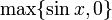$\max \{ \sin x, 0 \}$

The function also has the following description using a piecewise definition:$x \mapsto \left\lbrace \begin{array}{rl} \sin x, & 2n\pi \le x \le (2n + 1)\pi, n \in \mathbb{Z} \\ 0, & (2n - 1)\pi \le x \le 2n\pi, n \in mathbb{Z} \end{array}\right.$

Key data

Item Value
domain all real numbers, i.e., all of$\R$
range the closed interval$[0,1]$
absolute maximum value: 1, absolute minimum value: 0
period$2\pi$
local maximum values and points of attainment 1, at number of the form$2n\pi + \pi/2, n \in \mathbb{Z}$
local minimum values and points of attainment 0, at all points$x$ in intervals of the form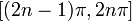$[(2n - 1)\pi,2n\pi]$, with$n \in \mathbb{Z}$.
first derivative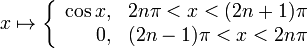$x \mapsto \left\lbrace \begin{array}{rl} \cos x, & 2n\pi < x < (2n + 1)\pi\\ 0, & (2n - 1)\pi < x < 2n\pi\end{array}\right.$
The function is not differentiable at integer multiples of$\pi$. At even multiples of$\pi$, the left hand derivative is 0 and the right hand derivative is 1. At odd multiples of$\pi$, the left hand derivative is -1 and the right hand derivative is 0.
second derivative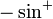$-\sin^+$, except at integer multiples of$\pi$, where it is undefined.
mean value over a period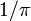$1/\pi$